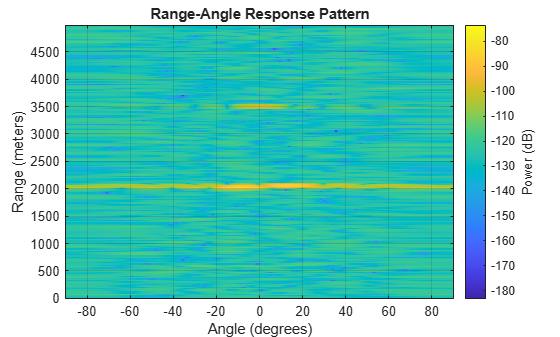# plotResponse

Plot range-angle response

Since R2018b

## Syntax

``plotResponse(response,X)``
``plotResponse(response,X,XREF)``
``plotResponse(response,X,COEFF)``
``plotResponse(___,'Unit',unit)``

## Description

````plotResponse(response,X)` plots the range response of a dechirped input signal, `X`, from the `phased.RangeAngleResponse` object, `response`. This syntax applies when you set the `RangeMethod` property to `'FFT'` and the `DechirpInput` property to `false`.```
````plotResponse(response,X,XREF)` plots the range response `X`, after performing a dechirp operation using the reference signal, `XREF`. This syntax applies when you set the `RangeMethod` property to `'FFT'` and the `DechirpInput` property to `true`.```
````plotResponse(response,X,COEFF)` plots the range response of `X` after match filtering using the match filter coefficients, `coeff`. This syntax applies when you set the `RangeMethod` property to ```'Matched filter'```.```

example

````plotResponse(___,'Unit',unit)` plots the response in the units specified by `units`.```

## Examples

collapse all

Calculate the range-angle response from a pulsed radar transmitting a rectangular waveform using the matched filter approach. The signal includes three target returns. Two are approximately 2000 m away and the third is approximately 3500 m away. In addition, two targets are stationary relative to the radar while the third is moving away from the radar at approximately 100 m/s. The signals arrive at an 8-element uniform linear array.

```load('RangeAngleResponseExampleData','rectdata'); fs = rectdata.fs; propspeed = rectdata.propspeed; fc = rectdata.fc; rxdata = rectdata.rxdata; mfcoeffs = rectdata.mfcoeffs; %noisepower = rectdata.noisepower; antennaarray = rectdata.antennaarray;```

Second, create the range-angle response object for matched filter processing.

```rngangresp = phased.RangeAngleResponse(... 'SensorArray',antennaarray,'OperatingFrequency',fc,... 'SampleRate',fs,'PropagationSpeed',propspeed);```

Obtain the range-angle map.

`[resp,rng_grid,ang_grid] = rngangresp(rxdata,mfcoeffs);`

Plot the response.

`plotResponse(rngangresp,rxdata,mfcoeffs,'Unit','db');`## Input Arguments

collapse all

Range-angle response object, specified as a `phased.RangeAngleResponse` System object.

Input data, specified as a complex-valued K-by-N matrix. The contents of the data cube depend on the type of range-angle processing specified by the different syntaxes. K always specifies the number of fast-time samples and N is always the number of channels, either array elements or beams.

• K is the number of fast-time or range samples.

• N is the number of independent spatial channels such as sensors or directions.

The size of the first dimension of the input matrix can vary to simulate a changing signal length. A size change can occur, for example, in the case of a pulse waveform with variable pulse repetition frequency.

Reference signal used for dechirping, specified as a complex-valued K-by-1 column vector. The number of rows must equal the length of the fast-time dimension of `X`.

#### Dependencies

To enable this input argument, set the value of `RangeMethod` to `'FFT'` and `DechirpInput` to `true`.

Data Types: `double`
Complex Number Support: Yes

Matched filter coefficients, specified as a complex-valued P-by-1 column vector. P must be less than or equal to K. K is the number of fast-time or range sample.

#### Dependencies

To enable this input argument, set the value of `RangeMethod` to ```'Matched filter'```.

Data Types: `double`
Complex Number Support: Yes

Plot units, specified as `'db'`, `'mag'`, or `'pow'`. who

• `'db'` – plot the response power in dB.

• `'mag'` – plot the magnitude of the response.

• `'pow'` – plot the response power.

Example: `'mag'`

Data Types: `char` | `string`

## Version History

Introduced in R2018b